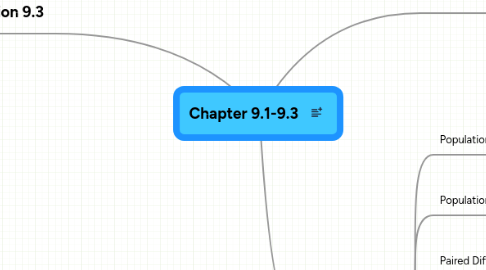Chapter 9.1-9.3

Get Started. It's FreeChapter 9.1-9.3 by2. Section 9.3

2.1. Sampling distribution

2.1.1. Is the probability distribution of possible values of the statistic for repeated samples of the same size taken from the same population.

2.2. Standard deviation of x

2.2.1. The standard deviation of the sampling distribution of a sample mean.

2.3. Standard deviation of p

2.3.1. The standard deviation of the sampling distribution of a sample proportion.

2.4. Standard error

2.4.1. describes the estimated standard deviation for a sampling distribution.

3. Section 9.2

3.1. Population Proportion

3.1.1. Which is a number between 0 and 1 representing the proportion with that trait.

3.2. Population mean

3.2.1. One of the simplest summaries for the population is the average of the variable for everyone in the population.

3.3. Paired Difference

3.3.1. Data that are formed by taking the differences in matched pairs.

3.4. Population mean for paired differences

3.4.1. Which is the mean that we would get if we took differences for the entire population of possible pairs.

3.5. Independent samples

3.5.1. The individuals in one sample are not coupled in any way with the individuals in the other sample.

3.6. Difference in two population means

3.6.1. The parameter of interest is the difference in two population means.